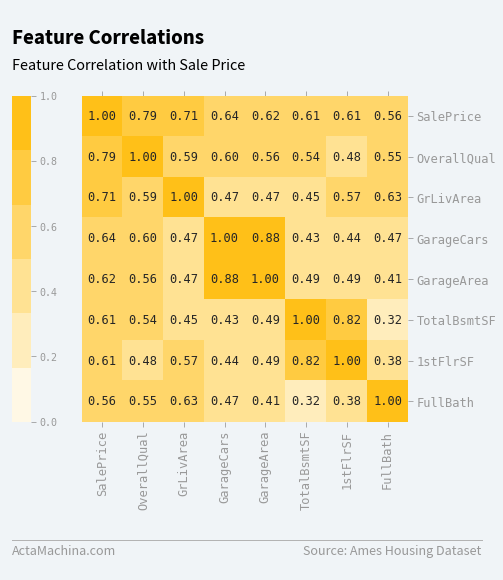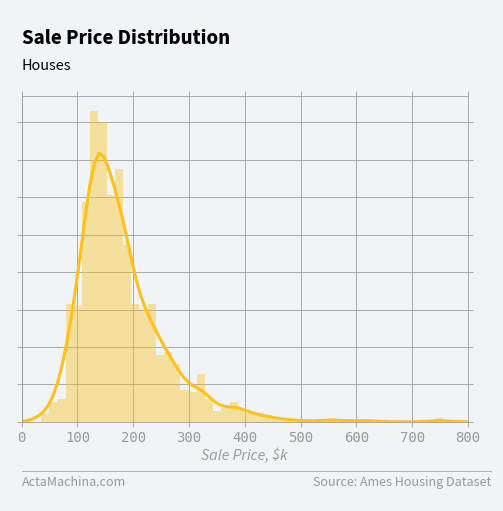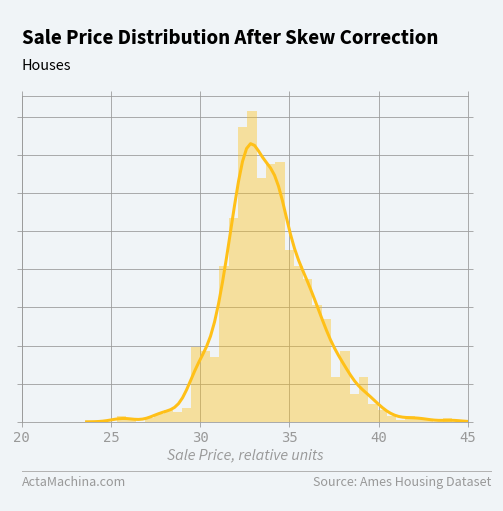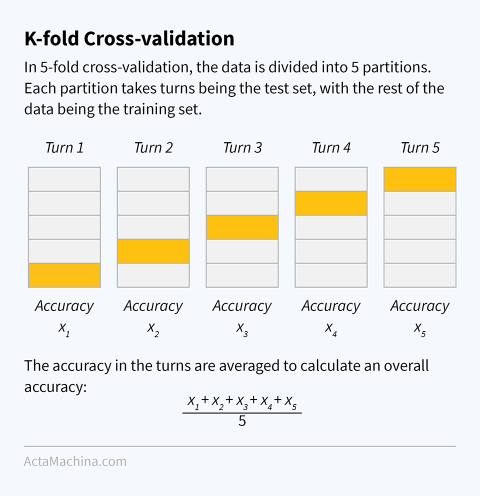This post introduces Machine Learning regression. Regression is the prediction of a quantity/number, in contrast with classification where a class is predicted. We will use the Ames Housing Dataset compiled by Dean De Cock to predict the sale price of houses in Ames, Iowa.

In this post, you'll learn how to:

• present data using the Seaborn data visualisation package;
• train and evaluate a regression model; and
• combine multiple models for a better result.

By the end, you'll learn how to predict the sale price of all 1,459 houses in test.csv. Here are a preview of the first 5 houses:

Out:
MSSubClass MSZoning LotFrontage LotArea Street Alley LotShape LandContour Utilities LotConfig ... ScreenPorch PoolArea PoolQC Fence MiscFeature MiscVal MoSold YrSold SaleType SaleCondition
Id
1461 20 RH 80.0 11622 Pave NaN Reg Lvl AllPub Inside ... 120 0 NaN MnPrv NaN 0 6 2010 WD Normal
1462 20 RL 81.0 14267 Pave NaN IR1 Lvl AllPub Corner ... 0 0 NaN NaN Gar2 12500 6 2010 WD Normal
1463 60 RL 74.0 13830 Pave NaN IR1 Lvl AllPub Inside ... 0 0 NaN MnPrv NaN 0 3 2010 WD Normal
1464 60 RL 78.0 9978 Pave NaN IR1 Lvl AllPub Inside ... 0 0 NaN NaN NaN 0 6 2010 WD Normal
1465 120 RL 43.0 5005 Pave NaN IR1 HLS AllPub Inside ... 144 0 NaN NaN NaN 0 1 2010 WD Normal

5 rows × 79 columns

## Import Packages¶

We'll be importing the same packages as the previous post, namely scipy, sklearn and pandas. We'll also include the data visualisation library seaborn since it contains some useful plots.

In :
from scipy.special import boxcox1p, inv_boxcox
from scipy.stats import skew

from pandas.api.types import CategoricalDtype

from sklearn.base import clone, BaseEstimator, RegressorMixin
from sklearn.ensemble import RandomForestRegressor
from sklearn.model_selection import cross_val_score, train_test_split
from sklearn.linear_model import Lasso, Ridge

import pandas as pd
import seaborn as sns


Let's load the training data in train.csv into a Pandas DataFrame with Id as index.

Unlike the previous post, where we only had one row to predict, we wish to predict all 1,459 rows inside test.csv. So, load the training and test data from two separate files into two DataFrames: df_train (1,460 rows) and df_test (1,459 rows). Any analysis and insights should only be obtained from the df_train and not be informed by df_test.

In :
df_train = pd.read_csv('data/house_prices/train.csv', index_col='Id')


Out:
MSSubClass MSZoning LotFrontage LotArea Street Alley LotShape LandContour Utilities LotConfig ... PoolArea PoolQC Fence MiscFeature MiscVal MoSold YrSold SaleType SaleCondition SalePrice
Id
1 60 RL 65.0 8450 Pave NaN Reg Lvl AllPub Inside ... 0 NaN NaN NaN 0 2 2008 WD Normal 208500
2 20 RL 80.0 9600 Pave NaN Reg Lvl AllPub FR2 ... 0 NaN NaN NaN 0 5 2007 WD Normal 181500
3 60 RL 68.0 11250 Pave NaN IR1 Lvl AllPub Inside ... 0 NaN NaN NaN 0 9 2008 WD Normal 223500
4 70 RL 60.0 9550 Pave NaN IR1 Lvl AllPub Corner ... 0 NaN NaN NaN 0 2 2006 WD Abnorml 140000
5 60 RL 84.0 14260 Pave NaN IR1 Lvl AllPub FR2 ... 0 NaN NaN NaN 0 12 2008 WD Normal 250000

5 rows × 80 columns

The DataFrame has 80 columns:

In :
df_train.info(verbose=False)

<class 'pandas.core.frame.DataFrame'>
Int64Index: 1460 entries, 1 to 1460
Columns: 80 entries, MSSubClass to SalePrice
dtypes: float64(3), int64(34), object(43)
memory usage: 923.9+ KB


Some of the features include SalePrice, MSSubClass, MSZoning and LotFrontage:

Feature Description
SalePrice Property's Sale Price in Dollars
MSSubClass Building Class
MSZoning General Zoning Classification
LotFrontage Linear Feet of Street Connected to Property
... ...

The data visualisation library, Seaborn, has lots of interesting plots like Hexbin, Kernel Density Estimate (KDE) and Violin plots.

This Heatmap plot shows the relationships of the 7 features most correlated with SalePrice:

In [ ]:
k = 8  # number of features for heatmap

correlation_matrix = df_train.corr()
columns = correlation_matrix.nlargest(k, 'SalePrice').SalePrice.index

covariance_matrix = np.corrcoef(df_train[columns].values.T)
sns.heatmap(covariance_matrix)From the Heatmap, you can see that the top 7 features most highly correlated with SalePrice are:

1. OverallQual: overall material and finish quality;
2. GrLivArea: above grade (ground) living area, in square feet;
3. GarageCars: size of garage, in car capacity;
4. GarageArea: size of garage, in square feet;
5. TotalBsmtSF: total square feet of basement area;
6. 1stFlrSF: size of first floor, in square feet; and
7. FullBath: full bathrooms, above grade.

## Data Pre-processing¶

The data pre-processing steps here are similar to the ones in the previous post. We want to:

• convert all columns to their correct data types, removing columns of object data type;
• impute (meaningfully fill in) or remove missing data;
• one-hot encode categories; and
• fix skewness for numerical features.

### Convert Columns to Correct Data Types¶

Columns of object data type are either actual strings or categories in the form of strings.

Let's check all the columns of object data type and see how many unique values they contain:

In :
df_train.select_dtypes(include='object').nunique().sort_values(ascending=False)

Out:
Neighborhood     25
Exterior2nd      16
Exterior1st      15
Condition1        9
SaleType          9
Condition2        8
HouseStyle        8
RoofMatl          8
Functional        7
SaleCondition     6
BsmtFinType2      6
Heating           6
Foundation        6
GarageType        6
RoofStyle         6
BsmtFinType1      6
ExterCond         5
BldgType          5
LotConfig         5
MSZoning          5
GarageCond        5
GarageQual        5
HeatingQC         5
Electrical        5
FireplaceQu       5
LotShape          4
LandContour       4
MiscFeature       4
Fence             4
BsmtExposure      4
BsmtCond          4
KitchenQual       4
MasVnrType        4
ExterQual         4
BsmtQual          4
GarageFinish      3
PavedDrive        3
PoolQC            3
LandSlope         3
Utilities         2
CentralAir        2
Alley             2
Street            2
dtype: int64

Since df_train has a total of 1,460 rows, we can assume that even the column with 25 unique values is a category column. So, we convert all columns of object data type into category:

In :
object_columns = df_train.select_dtypes(include='object').columns

for object_column in object_columns:
# ensure same categories across df_train and df_test
categories = set(pd.concat([df_train[object_column], df_test[object_column]]).dropna())

df_train[object_column] = df_train[object_column].astype(CategoricalDtype(categories=categories))
df_test[object_column] = df_test[object_column].astype(CategoricalDtype(categories=categories))


### Impute/Remove Missing Data¶

Let's create a missing data row count for each feature:

In :
missing_data = pd.DataFrame({'Count': df_train.isnull().sum(),
'MissingPercentage': df_train.isnull().sum() / df_train.isnull().count() * 100})

missing_data[missing_data.Count > 0].sort_values(by='Count', ascending=False)

Out:
Count MissingPercentage
PoolQC 1453 99.520548
MiscFeature 1406 96.301370
Alley 1369 93.767123
Fence 1179 80.753425
FireplaceQu 690 47.260274
LotFrontage 259 17.739726
GarageType 81 5.547945
GarageYrBlt 81 5.547945
GarageFinish 81 5.547945
GarageQual 81 5.547945
GarageCond 81 5.547945
BsmtExposure 38 2.602740
BsmtFinType2 38 2.602740
BsmtFinType1 37 2.534247
BsmtCond 37 2.534247
BsmtQual 37 2.534247
MasVnrArea 8 0.547945
MasVnrType 8 0.547945
Electrical 1 0.068493

We can remove features with missing data in more than 1% of its rows. Since we can't have any missing values after this step, if we don't remove the feature, we must remove the entire row instead. The key is to strike a balance between removing too many features and removing too many rows. The 1% figure is arbitrary but seems to be a good balance from observing the data.

In :
columns_to_remove = list(missing_data[missing_data.MissingPercentage > 1].index)
df_train.drop(columns_to_remove, axis=1, inplace=True)
df_test.drop(columns_to_remove, axis=1, inplace=True)


Now, we delete the remaining rows that contain missing values:

In :
df_train.dropna(inplace=True)
df_test.dropna(inplace=True)


### One-Hot Encoding¶

In :
df_train = pd.get_dummies(df_train)
df_test = pd.get_dummies(df_test)


### Fix Skewness for Numerical Features¶

Although we briefly covered the need to fix skewness for numerical features in the previous post, let's use Seaborn to plot the target feature, SalePrice as a distribution plot:

In [ ]:
sns.distplot(df_train.SalePrice);Now, we apply Box Cox transform to the features with skew > 0.75:

In :
lambda_ = 0.15

skewness = pd.DataFrame({'Skew': df_train.apply(lambda x: skew(x.dropna())).sort_values(ascending=False)})
skewed_columns = skewness[abs(skewness) > 0.75].dropna().index

print(f"There are {skewed_columns.shape} skewed features to Box Cox transform.")

for column in skewed_columns:
df_train[column] = boxcox1p(df_train[column], lambda_)

if column != 'SalePrice':
df_test[column] = boxcox1p(df_test[column], lambda_)

There are 199 skewed features to Box Cox transform.


Let's look at a distribution plot of SalePrice again after skew correction:

In [ ]:
sns.distplot(df_train.SalePrice);Now the features are ready for the machine learning regression model!

## Model¶

To prepare the data for the models, we separate the features (X) from the target label (y):

In :
X = df_train.drop('SalePrice', axis=1)
y = df_train.SalePrice.values.ravel()


First, we'll go through a simple regression model that is similar to the classification model in the previous post. Then, we'll delve into a more complicated ensemble model.

### Simple Model¶

In the previous post, we used train_test_split to split X and y into a train/test set before fitting and scoring the model. We can get a more reliable score by using the cross_val_score function to do this multiple times (on a different train/test split each time) and take the average. This is called $k$-fold cross-validation, where $k$ is the number of train/test splits. The value of $k$ can range from 2 (a single train/test split) to $N$, the total number of rows in the data set. The extreme case where $k = N$ is also called leave-one-out cross-validation.

Out:Here, we use the 5-fold cross-validation (where $k = 5$) to evaluate the model:

In :
model = RandomForestRegressor()
scores = cross_val_score(model, X, y, cv=5, scoring='r2')
print('Score: {:.5f} ({:.5f})'.format(scores.mean(), scores.std()))

Score: 0.85688 (0.02040)


Now let's train the model using all of X and y and then use the trained model to make predictions on df_test:

In :
model = RandomForestRegressor()
model.fit(X, y)
pred = model.predict(df_test)
pred

Out:
array([32.0262467 , 32.96734321, 33.97662815, ..., 33.25564396,
31.821167  , 35.92025014])

Wait, these predicted sale prices don't look quite right. Since we applied Box-Cox transform to help reshape SalePrice, we need to undo it by calling inv_boxcox:

In :
inv_boxcox(pred, lambda_)

Out:
array([123448.15398991, 144897.80806527, 171343.55636578, ...,
152070.92600908, 119151.13227291, 233946.41203722])
In :
pd.read_csv('data/house_prices/test.csv', index_col='Id').head()

Out:
MSSubClass MSZoning LotFrontage LotArea Street Alley LotShape LandContour Utilities LotConfig ... ScreenPorch PoolArea PoolQC Fence MiscFeature MiscVal MoSold YrSold SaleType SaleCondition
Id
1461 20 RH 80.0 11622 Pave NaN Reg Lvl AllPub Inside ... 120 0 NaN MnPrv NaN 0 6 2010 WD Normal
1462 20 RL 81.0 14267 Pave NaN IR1 Lvl AllPub Corner ... 0 0 NaN NaN Gar2 12500 6 2010 WD Normal
1463 60 RL 74.0 13830 Pave NaN IR1 Lvl AllPub Inside ... 0 0 NaN MnPrv NaN 0 3 2010 WD Normal
1464 60 RL 78.0 9978 Pave NaN IR1 Lvl AllPub Inside ... 0 0 NaN NaN NaN 0 6 2010 WD Normal
1465 120 RL 43.0 5005 Pave NaN IR1 HLS AllPub Inside ... 144 0 NaN NaN NaN 0 1 2010 WD Normal

5 rows × 79 columns

So, the first 5 houses of df_test (above) have predicted SalePrice of:

In :
inv_boxcox(pred[:5], lambda_)

Out:
array([123448.15398991, 144897.80806527, 171343.55636578, 186487.2081506 ,
206409.9966901 ])

### Ensemble Model¶

An ensemble model is one that combines the strengths of several simpler base models. There are three common types of ensemble models:

• boosting: uses multiple, identical models that are trained in sequence, with each subsequent model trained emphasising the mis-classifications of the previous model;
• bootstrap aggregation (or bagging): uses multiple, identical models that are trained on randomly drawn subsets of the training set; and
• stacking: uses different models to train the data.

Actually, the RandomForestClassifier and RandomForestRegressor which you've already been using are also ensemble models since they combine multiple decision trees using majority voting, with each decision tree generated using a random subset of features.

Here is an example of stacking. We create an instance of a StackedRegressor that combines the Lasso, RandomForestRegressor and Ridge regression models for a better prediction:

In :
class StackedRegressor(BaseEstimator, RegressorMixin):
def __init__(self, models):
self.models = models

def fit(self, X, y):
self.models_ = [clone(model) for model in self.models]

for model in self.models_:
model.fit(X, y)

return self

def predict(self, X):
predictions = np.column_stack([model.predict(X) for model in self.models_])
return np.mean(predictions, axis=1)

stacked_model = StackedRegressor([
Lasso(),
RandomForestRegressor(),
Ridge(),
])

scores = cross_val_score(stacked_model, X, y, cv=5, scoring='r2')
print('Score: {:.5f} ({:.5f})'.format(scores.mean(), scores.std()))

Score: 0.86763 (0.02538)


We see that the StackedRegressor (86.763%) performed better than the RandomForestRegressor by itself (85.688%).

## Summary and Next Steps¶

In this post, you've learnt how to:

• present data using the Seaborn data visualisation package;
• train and evaluate a regression model; and
• combine multiple models for a better result.

Now you're familar with both types of Supervised Learning: classification (predicting a class) and regression (predicting a quantity). There are two other broad types of Machine Learning: (i) Unsupervised Learning is when you are only given inputs and tasked to find interesting attributes of the dataset, such as clusters or outliers; and (ii) Reinforcement Learning is where an agent interacts with an environment and changes its action based on rewards. These will be explored in future posts. Stay tuned!

## Acknowledgements¶

This post has been inspired by posts from: